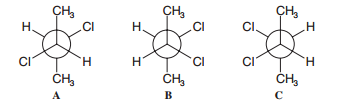# Problem: Shown below are Newman projection formulas for (R,R)-, (S,S)-, and (R,S)-2,3-dichlorobutane. (a) Which is (R,R)-?

🤓 Based on our data, we think this question is relevant for Professor Fatima's class at UWATERLOO.

###### Problem Details

Shown below are Newman projection formulas for (R,R)-, (S,S)-, and (R,S)-2,3-dichlorobutane. (a) Which is (R,R)-?What scientific concept do you need to know in order to solve this problem?

Our tutors have indicated that to solve this problem you will need to apply the R and S Configuration concept. You can view video lessons to learn R and S Configuration. Or if you need more R and S Configuration practice, you can also practice R and S Configuration practice problems.

What is the difficulty of this problem?

Our tutors rated the difficulty ofShown below are Newman projection formulas for (R,R)-, (S,S)...as high difficulty.

How long does this problem take to solve?

Our expert Organic tutor, Jonathan took 5 minutes and 24 seconds to solve this problem. You can follow their steps in the video explanation above.

What professor is this problem relevant for?

Based on our data, we think this problem is relevant for Professor Fatima's class at UWATERLOO.

What textbook is this problem found in?

Our data indicates that this problem or a close variation was asked in Organic Chemistry - Solomons 10th Edition. You can also practice Organic Chemistry - Solomons 10th Edition practice problems.